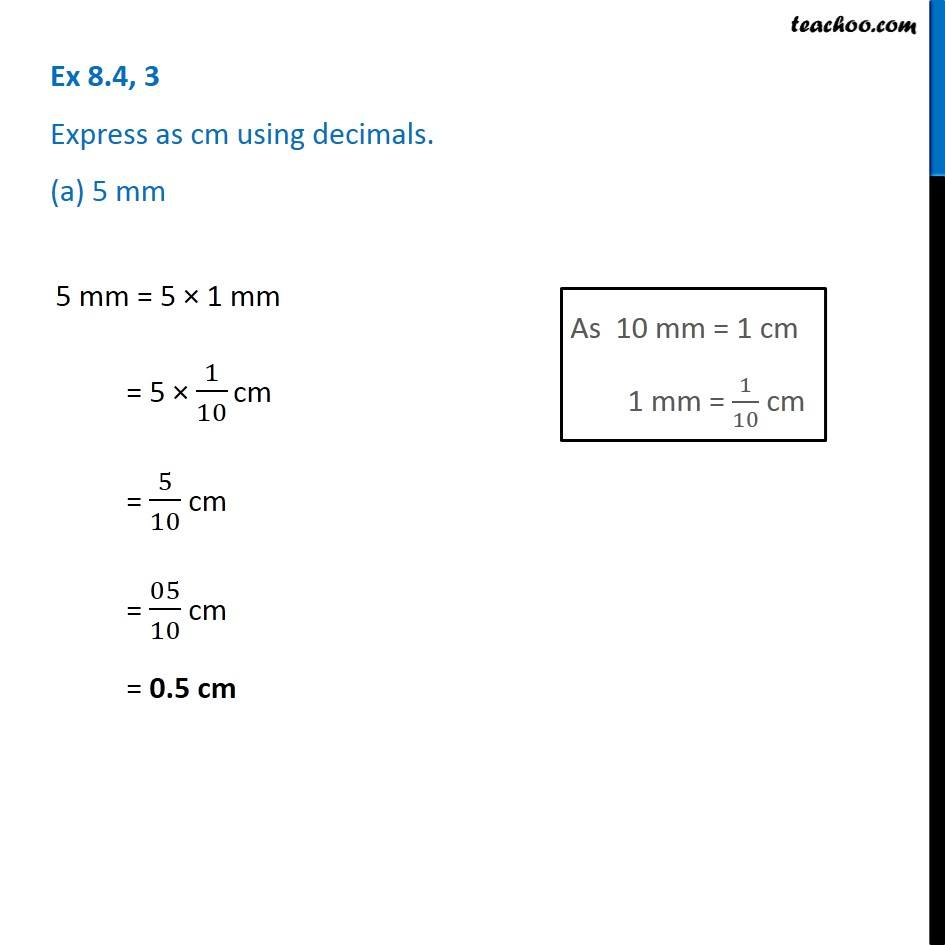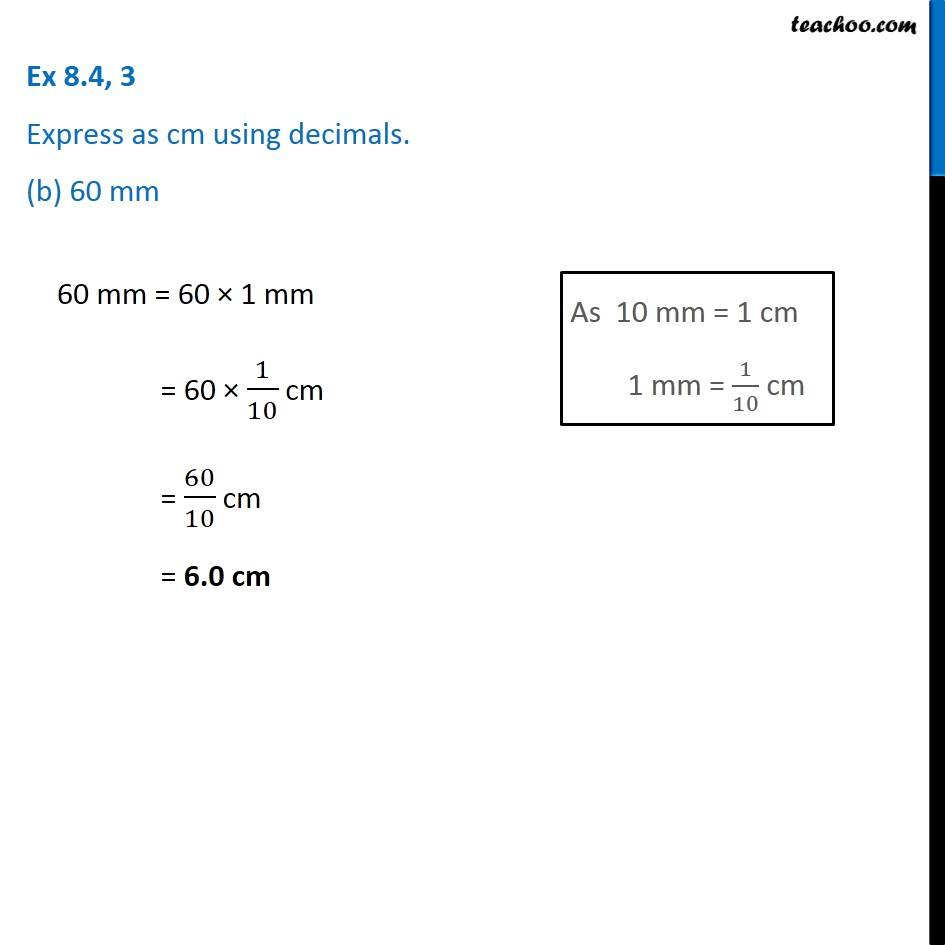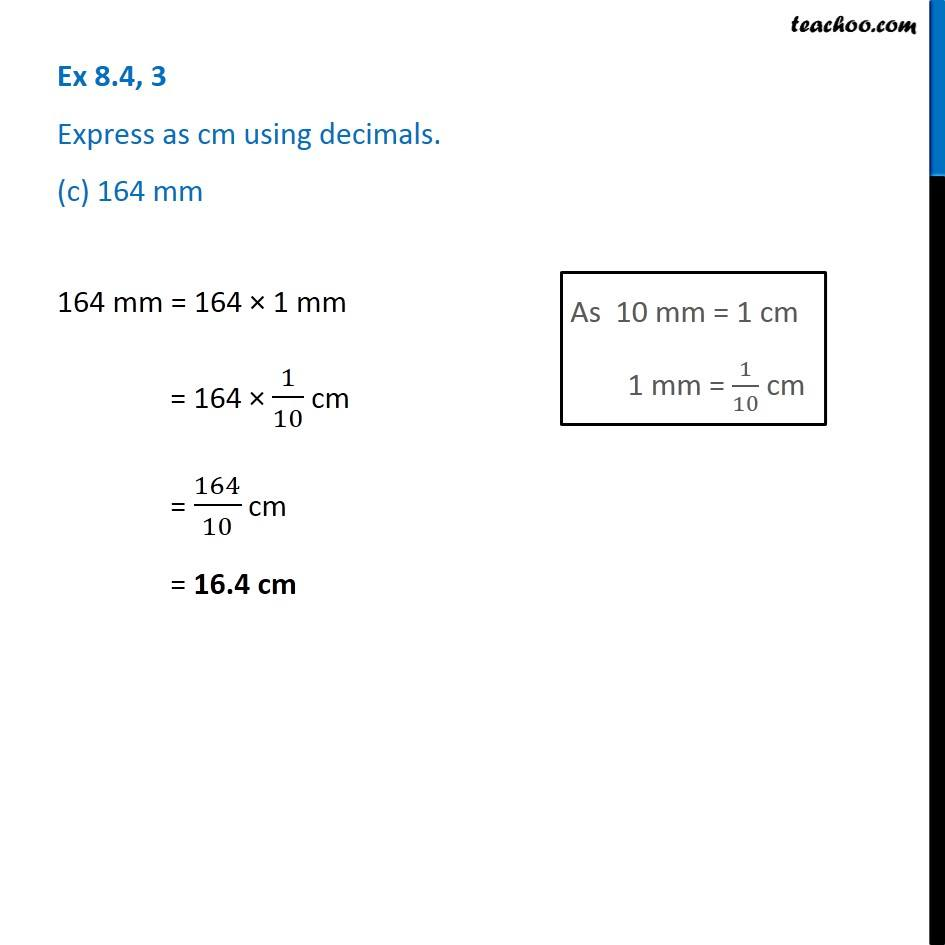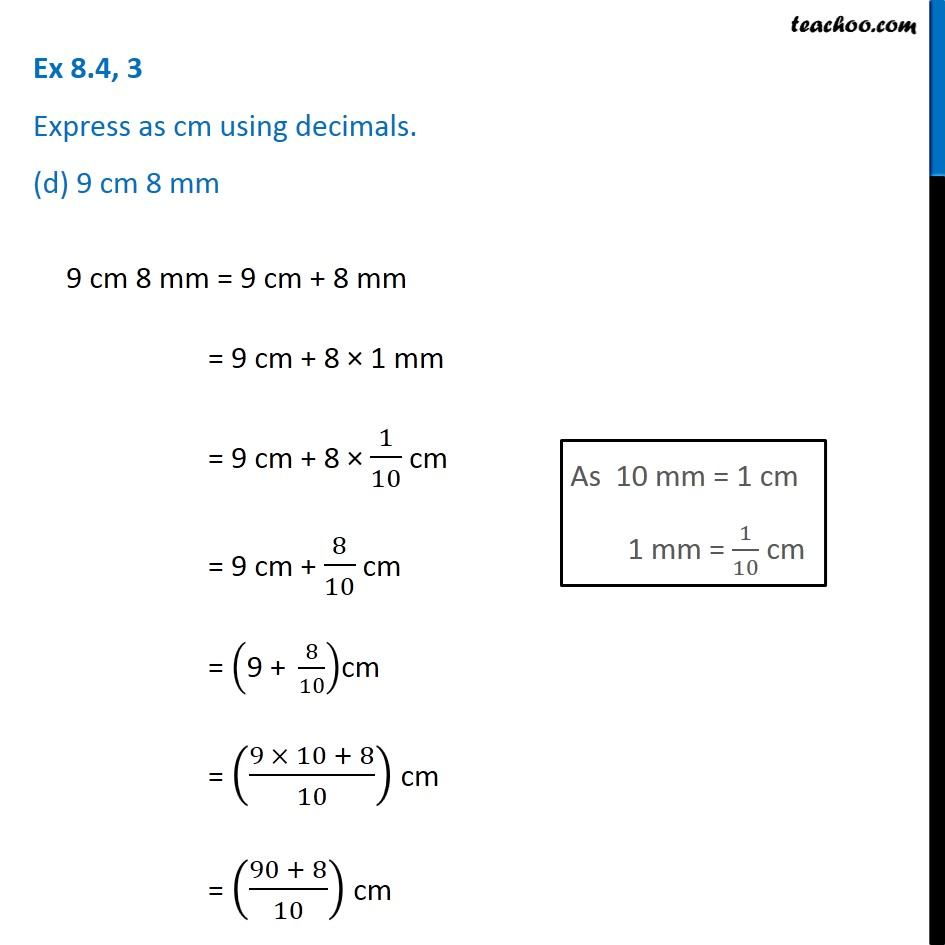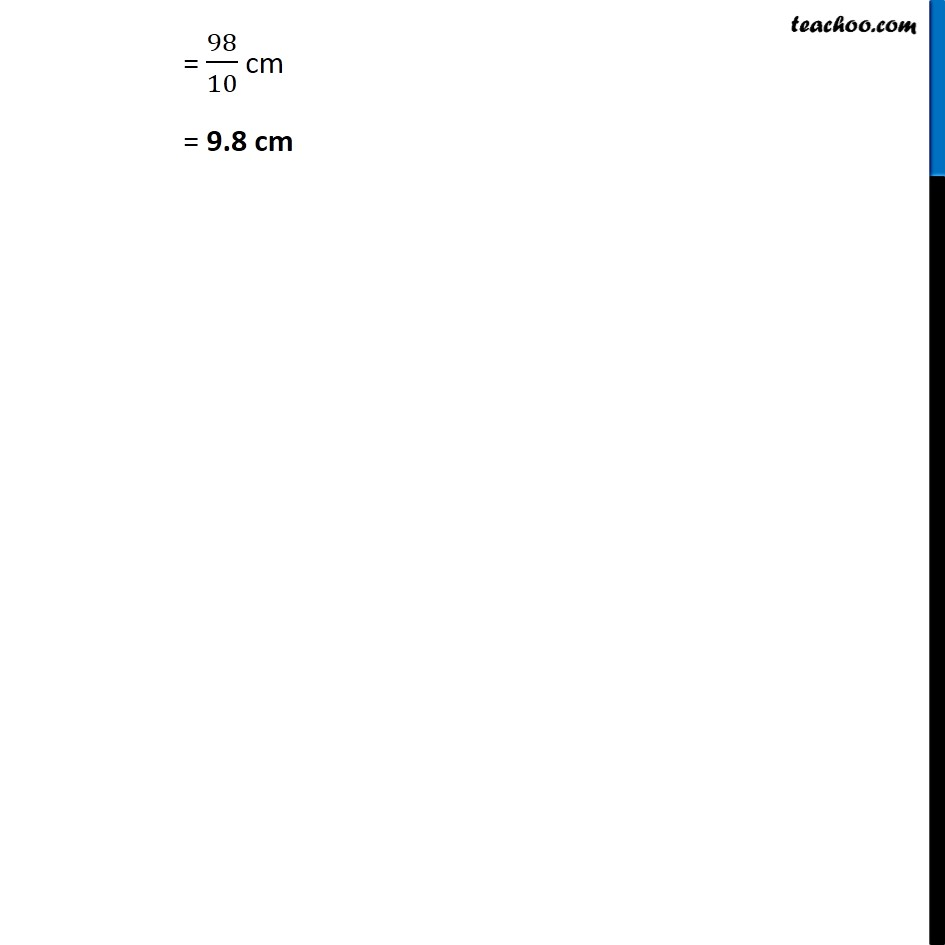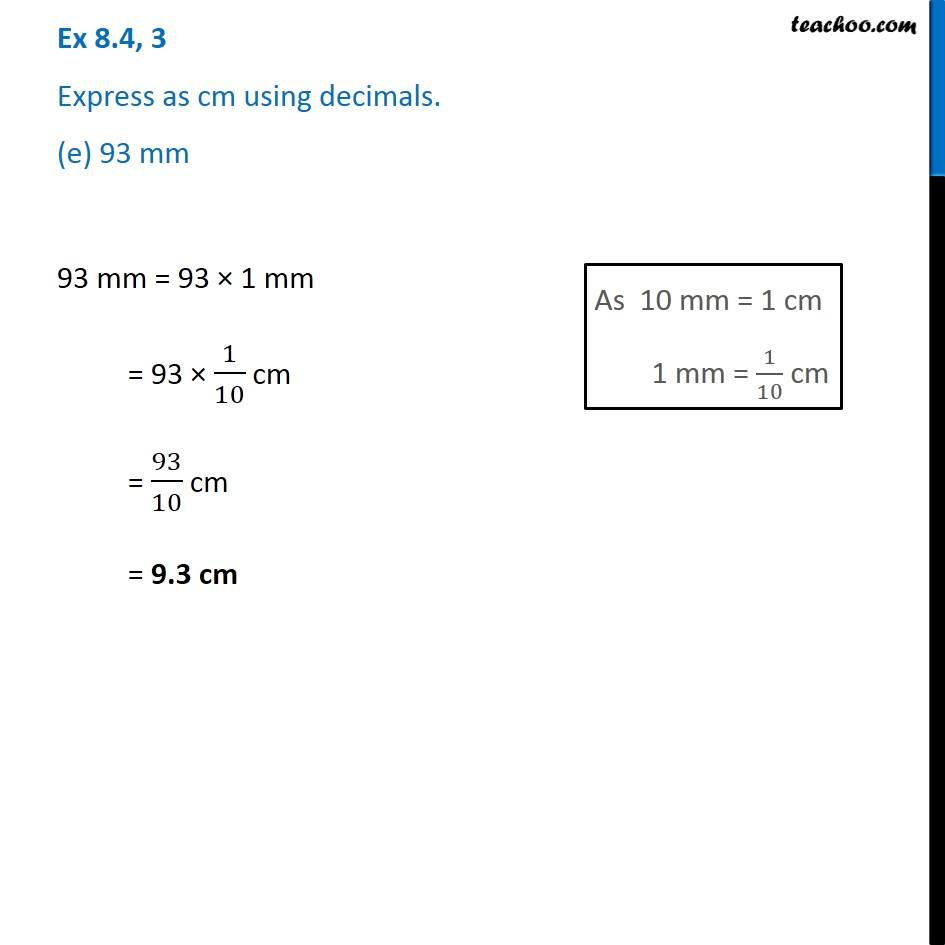1. Chapter 8 Class 6 Decimals
2. Serial order wise
3. Ex 8.4

Transcript

Ex 8.4, 3 Express as cm using decimals. (a) 5 mm 5 mm = 5 × 1 mm = 5 × 1/10 cm = 5/10 cm = 05/10 cm = 0.5 cm As 10 mm = 1 cm 1 mm = 1/10 cm Ex 8.4, 3 Express as cm using decimals. (b) 60 mm 60 mm = 60 × 1 mm = 60 × 1/10 cm = 60/10 cm = 6.0 cm As 10 mm = 1 cm 1 mm = 1/10 cm Ex 8.4, 3 Express as cm using decimals. (c) 164 mm 164 mm = 164 × 1 mm = 164 × 1/10 cm = 164/10 cm = 16.4 cm Ex 8.4, 3 Express as cm using decimals. (d) 9 cm 8 mm 9 cm 8 mm = 9 cm + 8 mm = 9 cm + 8 × 1 mm = 9 cm + 8 × 1/10 cm = 9 cm + 8/10 cm = ("9 + " 8/10)cm = ((9 × 10 + 8)/10) cm = ((90 + 8)/10) cm Ex 8.4, 3 Express as cm using decimals. (e) 93 mm Ex 8.4, 3 Express as cm using decimals. (e) 93 mm

Ex 8.4

Chapter 8 Class 6 Decimals
Serial order wise